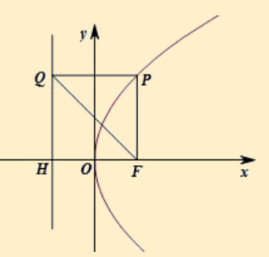$\text{A.}$ 3 $\text{B.}$ 6 $\text{C.}$ 9 $\text{D.}$ 12

B

#### 解析：

【解析】依题意 $\angle Q F H=\frac{\pi}{3} ， H F=3 ， Q H=3 \sqrt{3} ， Q F=6$ ，又 $P F=Q P$ ， $\angle P Q F=\frac{\pi}{2}$ ，则 $\triangle P Q F$ 为等边三角形，有 $P F=6$ ，选 : B.①点击 收藏 此题， 扫码注册关注公众号接收信息推送（一月四份试卷,中1+高2+研1）
② 程序开发、服务器资源都需要大量的钱，如果你感觉本站好或者受到到帮助，欢迎赞助本站,赞助方式：微信/支付宝转账到 18155261033

 ①此题难易度如何 ②此题推荐度如何 确定